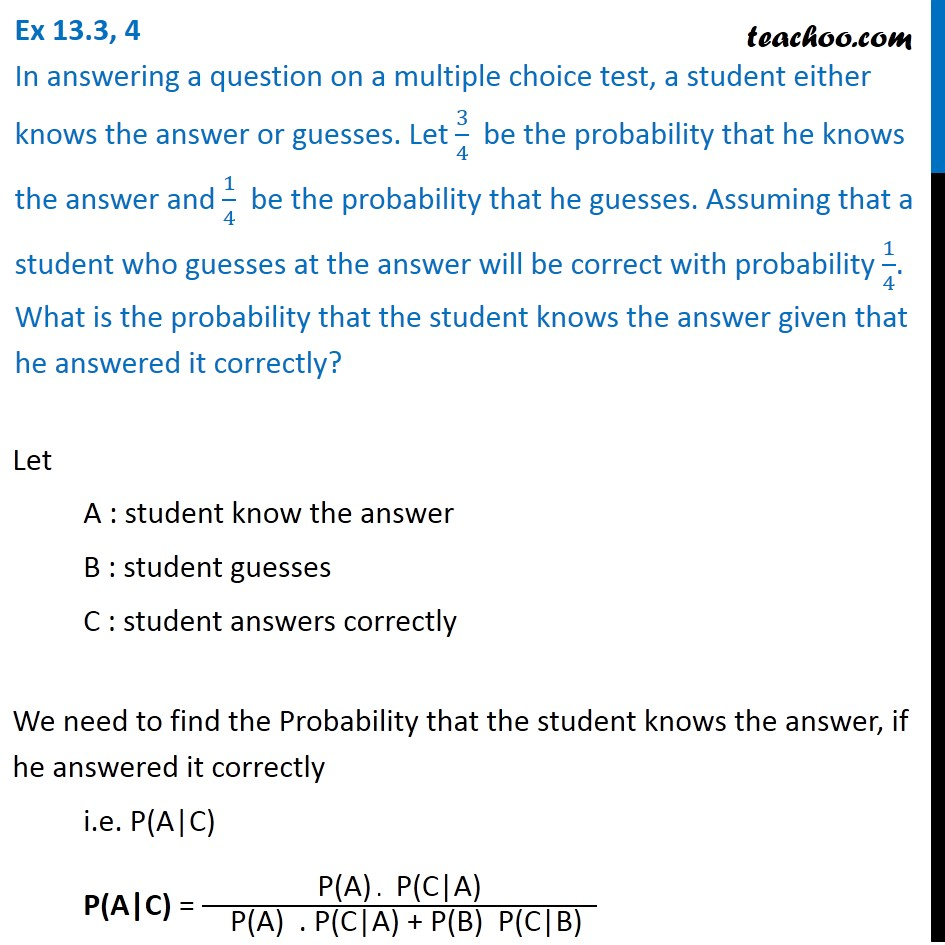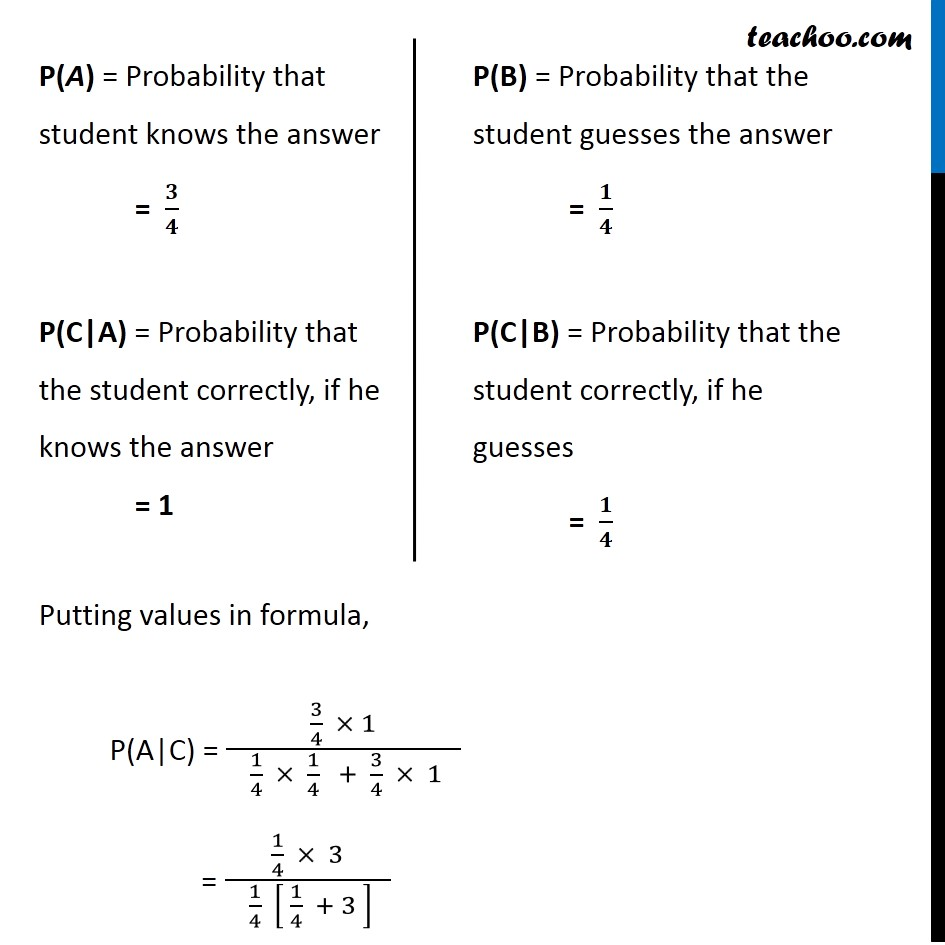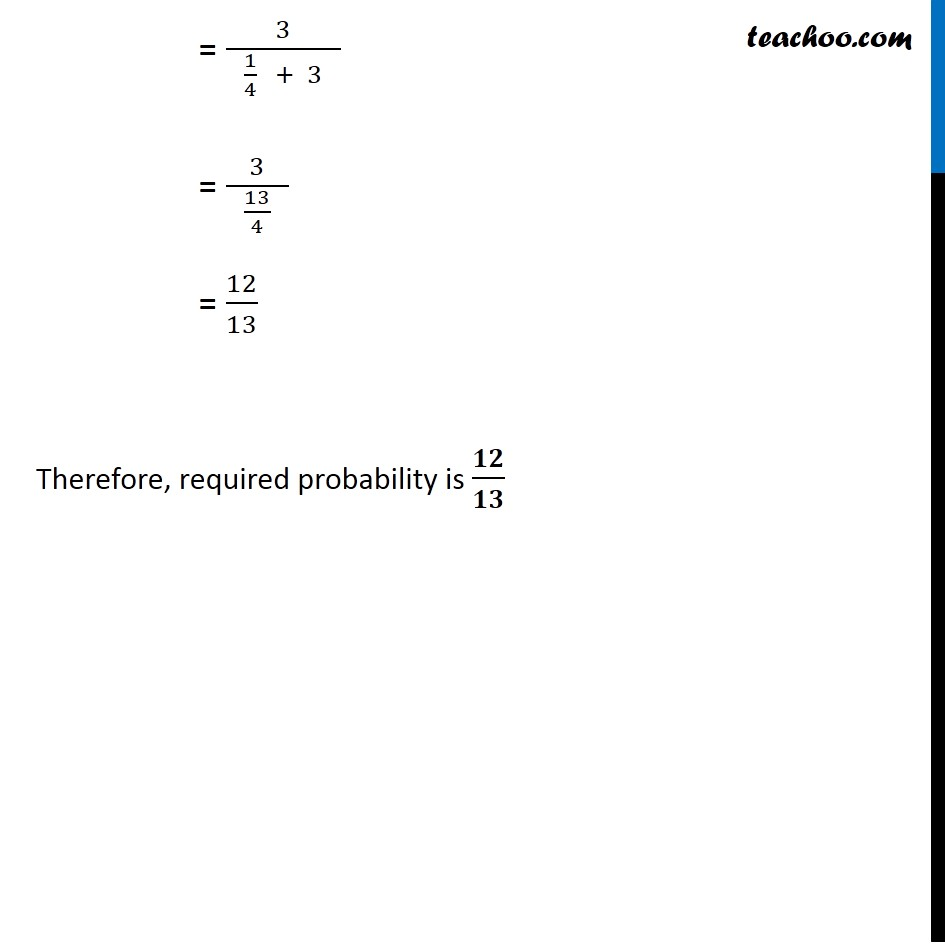Ex 13.3

Chapter 13 Class 12 Probability
Serial order wiseSolve all your doubts with Teachoo Black (new monthly pack available now!)

### Transcript

Ex 13.3, 4 In answering a question on a multiple choice test, a student either knows the answer or guesses. Let 3/4 be the probability that he knows the answer and 1/4 be the probability that he guesses. Assuming that a student who guesses at the answer will be correct with probability 1/4. What is the probability that the student knows the answer given that he answered it correctly?Let A : student know the answer B : student guesses C : student answers correctly We need to find the Probability that the student knows the answer, if he answered it correctly i.e. P(A|C) P(A|C) = ("P(A)" ." P(C|A)" )/" P(A) . P(C|A) + P(B) P(C|B) " "P(A)" = Probability that student knows the answer = 𝟑/𝟒 "P(C|A)" = Probability that the student correctly, if he knows the answer = 1 "P(B)" = Probability that the student guesses the answer = 𝟏/𝟒 "P(C|B)" = Probability that the student correctly, if he guesses = 𝟏/𝟒 Putting values in formula, P(A|C) = (3/4 × 1)/( 1/4 × 1/4 + 3/4 × 1 ) = (1/4 × 3)/( 1/4 [ 1/4 + 3 ] ) = 3/( 1/4 + 3 ) = 3/( 13/4 ) = 12/13 Therefore, required probability is 𝟏𝟐/𝟏𝟑